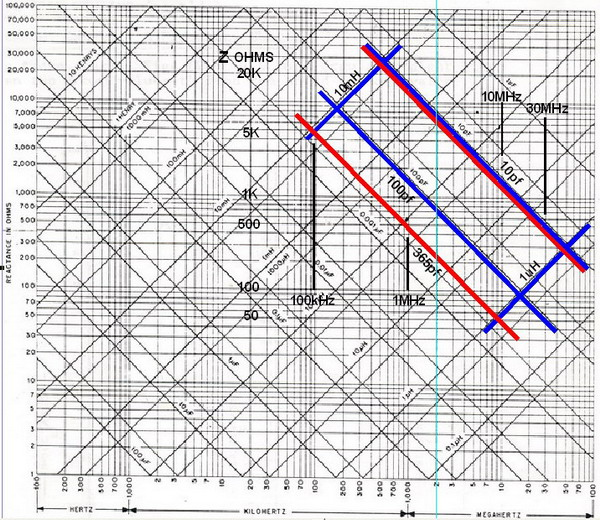Basic PRESELECTOR Technology This page has been added to offer a visual aid for understanding the inverse qualities of both inductive and capacitive reactances in relation to their respective component values. The graph below is a single picture that would otherwise require paragraphs of text to describe. Note the component values for the more ideal capacitor indicated by the blue box, (for the capacitor limited to 100pf) and the red box values for the more commonly used 365pf capacitors. Also note the Impedance values (Ohms) and how the utilization of low value capacitance and high value inductance renders a resonant combination of the highest impedance, when compared to other combinations with the reverse conditions. Before attempting to design, modify, or analyze a band pass or band stop filter, it is absolutely necessary to know and understand these few simple rules. Read them and refer to the graph until they are second nature. - The ratio of capacitance to capacitive reactance is inverse. That is, for a given frequency, as capacity goes up, reactance goes down.- The ratio of inductance to inductive reactance is proportional. That is, for a given frequency, as inductance goes up, reactance goes up.- In filter circuits, there are two forms of Q (efficiency): Component Q, and filter Q.     - Component Q is the quality of the device: Reactance/Resistance, Z/R.     - Filter Q is the efficiency of the filter loop: ratio of the loop impedance to the filter impedance.- Series tuned resonant circuits are at minimum impedance at resonance.- The efficiency of series tuned filters increases as the circuit (loop) impedance decreases.- Parallel tuned resonant circuits are at maximum impedance at resonance.- The efficiency of parallel tuned filters increases as the circuit (loop) impedance increases.Example of component selection:Let's assume a filter design for a single center frequency of 1 MHz. Follow the 1 MHz line vertically. Note that with the 10-365pf capacitor (red), inductors of from 100 uH to 2 mH will resonate at the design frequency. Note the highest impedance is when the cap is at the lowest value. Also note that at the capacitor's greatest value, the filter's impedance is only about 500 Ohms and at its lowest value the filter impedance is about 15,000 Ohms!Now look at the smaller capacitor (blue) and see that the impedance range of the filter is 1,500 to 15,000 Ohms. It becomes quite obvious that using a variable tuning cap with a value range that is restricted to about 10:1 is the better choice for a high-Q filter circuit. It is also apparent that the lowest value that the variable cap is capable of, should be selected based upon the band of frequencies of interest and the availability of suitable high-Q inductors of complementary value.Please read: Preselector Design and Theory Home18 Aug 2006
Hosted by www.Geocities.ws